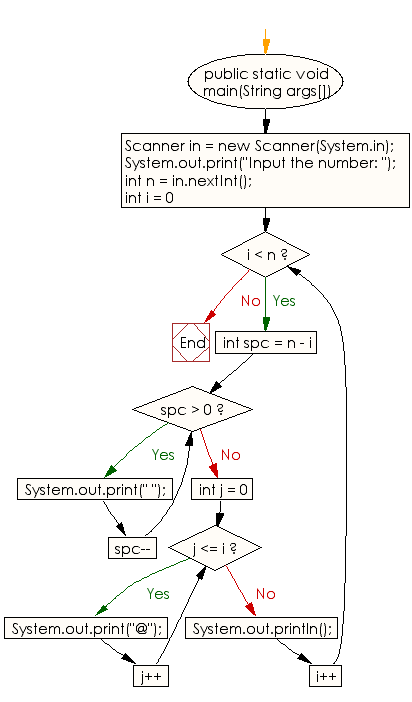﻿ Java exercises: Generate a following @'s triangle - w3resource# Java Conditional Statement Exercises: Generate a following @'s triangle

## Java Conditional Statement: Exercise-24 with Solution

Write a java program to generate a following @'s triangle.

Test Data
Input the number: 6

Pictorial Presentation:Sample Solution:

Java Code:

``````import java.util.Scanner;
public class Exercise24 {
public static void main(String args[])
{
Scanner in = new Scanner(System.in);
System.out.print("Input the number: ");
int n = in.nextInt();

for (int i = 0; i < n; i++)
{
for (int spc = n - i; spc > 0; spc--)
{
System.out.print(" ");
}
for (int j = 0; j <= i; j++)
{
System.out.print("@");
}
System.out.println();
}
}

}
```
```

Sample Output:

```Input the number: 6
@
@@
@@@
@@@@
@@@@@
@@@@@@
```

Flowchart:Java Code Editor:

What is the difficulty level of this exercise?

﻿

## Java: Tips of the Day

How to split a String by space?

You can use the whitespace regex:

```str = "Hello I'm your String";
String[] splited = str.split("\\s+");
```

This will cause any number of consecutive spaces to split your string into tokens.

Ref: https://bit.ly/3xk42If